# Repeated use of integration by parts

## In an equivalence of integration problems language

Integration by parts can be used multiple times, i.e., the new integration that we obtain from an application of integration by parts can again be subjected to integration by parts. However, we need to make sure that we avoid the circular trap.

The typical repeated application of integration by parts looks like:$F(x)g(x) \to F'(x)\int g(x) \, dx \to F''(x) \int \int g(x) \, dx \, dx \to \dots$

In words, we keep differentiating the part obtained by differentiation and keep integrating the part obtained by integration. Note that after$k$ applications of the procedure, we would have a product of the$k^{th}$ derivative of$F$ and a$k^{th}$ antiderivative of$g$.

## How the expressions actually look

### Case of integration by parts applied twice

Consider:$\int F(x)g(x) \, dx$

Suppose$q$ is a function whose second derivative is$g$, so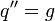$q'' = g$. Then, we have: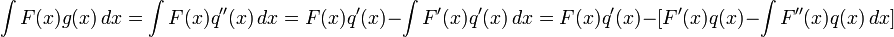$\int F(x)g(x)\, dx = \int F(x)q''(x) \, dx = F(x)q'(x) - \int F'(x)q'(x) \, dx = F(x)q'(x) - [F'(x)q(x) - \int F''(x)q(x) \, dx]$

Simplify, we get: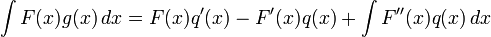$\int F(x)g(x)\, dx = F(x)q'(x) - F'(x)q(x) + \int F''(x)q(x) \, dx$

Note once again the integrals are not equal. As we see here, the actual application of integration by parts will yield a number of other terms with alternating signs. What we are saying is that the original integration problem has been reduced to a new integration problem where one factor of the product has been repeatedly differentiated and the other factor has been repeatedly integrated.

### Case of integration by parts applied$k$ times

Consider:$\int F(x)g(x) \, dx$

Suppose$q$ is a function whose$k^{th}$ derivative is$g$, so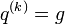$q^{(k)} = g$. Then, we have:$\int F(x)g(x)\, dx = \int F(x)q^{(k)}(x) \, dx = F(x)q^{(k-1)}(x) - \int F'(x)q^{(k-1)}(x) \, dx = \dots$

After repeated application, we finally get: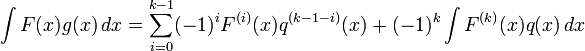$\int F(x)g(x)\, dx = \sum_{i=0}^{k-1} (-1)^i F^{(i)}(x)q^{(k-1-i)}(x) + (-1)^k \int F^{(k)}(x)q(x) \, dx$

In particular, there are$k$ products outside the integral symbol, with a new one being added for every application of integration by parts.

Note that we need to do a total of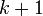$k + 1$ integrations: integrate$g$$k$ times to get$q$, and then do the final integration in the expression on the right side.

## Examples

### Polynomial times trigonometric or exponential

When trying to integrate the product of a polynomial function of degree$d$ and a trigonometric or exponential function, we need to apply integration by parts$d$ times, taking the polynomial as the part to differentiate each time. The trigonometric or exponential piece ends up getting integrated a total of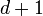$d + 1$ times.